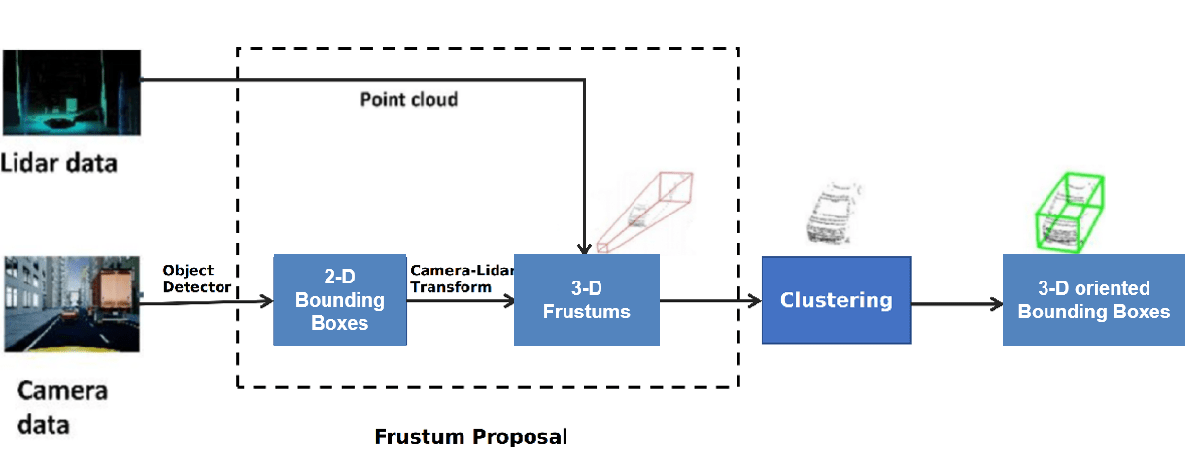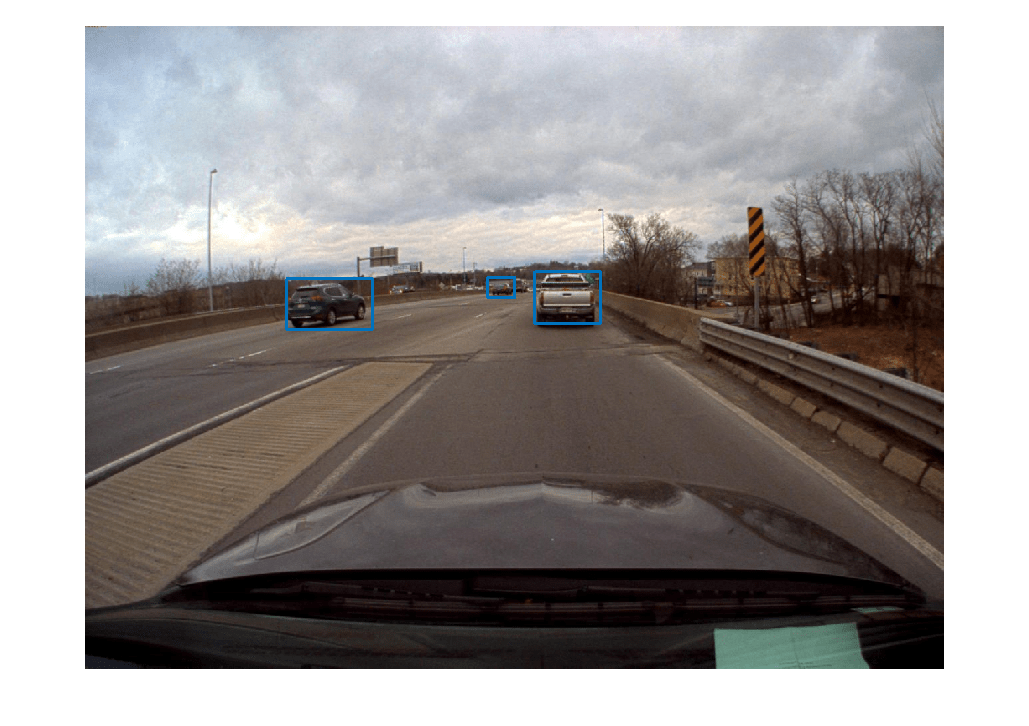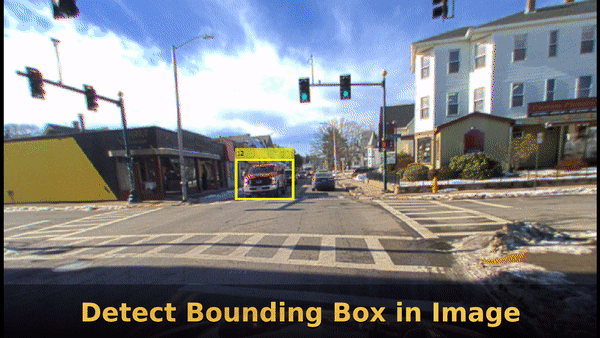# Detect Vehicles in Lidar Using Image Labels

This example shows you how to detect vehicles in lidar using label data from a co-located camera with known lidar-to-camera calibration parameters. Use this workflow in MATLAB® to estimate 3-D oriented bounding boxes in lidar based on 2-D bounding boxes in the corresponding image. You will also see how to automatically generate ground truth as a distance for 2-D bounding boxes in a camera image using lidar data. This figure provides an overview of the process.This example uses lidar data collected on a highway from an Ouster OS1 lidar sensor and image data from a front-facing camera mounted on the ego vehicle. The lidar and camera data are approximately time-synced and calibrated to estimate their intrinsic and extrinsic parameters. For more information on lidar camera calibration, see Lidar and Camera Calibration.

Note: The download time for the data depends on the speed of your internet connection. During the execution of this code block, MATLAB is temporarily unresponsive.

```lidarTarFileUrl = 'https://www.mathworks.com/supportfiles/lidar/data/WPI_LidarData.tar.gz'; imageTarFileUrl = 'https://www.mathworks.com/supportfiles/lidar/data/WPI_ImageData.tar.gz'; outputFolder = fullfile(tempdir,'WPI'); lidarDataTarFile = fullfile(outputFolder,'WPI_LidarData.tar.gz'); imageDataTarFile = fullfile(outputFolder,'WPI_ImageData.tar.gz'); if ~exist(outputFolder,'dir') mkdir(outputFolder) end if ~exist(lidarDataTarFile,'file') disp('Downloading WPI Lidar driving data (760 MB)...') websave(lidarDataTarFile,lidarTarFileUrl) untar(lidarDataTarFile,outputFolder) end % Check if lidar tar.gz file is downloaded, but not uncompressed. if ~exist(fullfile(outputFolder,'WPI_LidarData.mat'),'file') untar(lidarDataTarFile,outputFolder) end if ~exist(imageDataTarFile,'file') disp('Downloading WPI Image driving data (225 MB)...') websave(imageDataTarFile,imageTarFileUrl) untar(imageDataTarFile,outputFolder) end % Check if image tar.gz file is downloaded, but not uncompressed. if ~exist(fullfile(outputFolder,'imageData'),'dir') untar(imageDataTarFile,outputFolder) end imageDataLocation = fullfile(outputFolder,'imageData'); images = imageSet(imageDataLocation); imageFileNames = images.ImageLocation; % Load downloaded lidar data into the workspace lidarData = fullfile(outputFolder,'WPI_LidarData.mat'); load(lidarData); % Load calibration data if ~exist('calib','var') load('calib.mat') end % Define camera to lidar transformation matrix camToLidar = calib.extrinsics; intrinsics = calib.intrinsics;```

This example uses prelabeled data to serve as ground truth for the 2-D detections from the camera images. These 2-D detections can be generated using deep learning-based object detectors like `vehicleDetectorYOLOv2`, `vehicleDetectorFasterRCNN`, and `vehicleDetectorACF`. For this example, the 2-D detections have been generated using the Image Labeler app. These 2-D bounding boxes are vectors of the form: $\left[\mathit{x}\text{\hspace{0.17em}}\mathit{y}\text{\hspace{0.17em}}\mathit{w}\text{\hspace{0.17em}}\mathit{h}\right]$, where $\mathit{x}\text{\hspace{0.17em}}\mathrm{and}\text{\hspace{0.17em}}\mathit{y}$ represent the xy-coordinates of the top-left corner, and $\mathit{w}\text{\hspace{0.17em}}\mathrm{and}\text{\hspace{0.17em}}\mathit{h}$ represent the width and height of the bounding box respectively.

Read a image frame into the workspace, and display it with the bounding boxes overlaid.

```load imageGTruth.mat im = imread(imageFileNames{50}); imBbox = imageGTruth{50}; figure imshow(im) showShape('rectangle',imBbox)```### 3-D Region Proposal

To generate cuboid bounding boxes in lidar from the 2-D rectangular bounding boxes in the image data, a 3-D region is proposed to reduce the search space for bounding box estimation. The corners of each 2-D rectangular bounding box in the image are transformed into 3-D lines using camera intrinsic parameters and camera-to-lidar extrinsic parameters. These 3-D lines form frustum flaring out from the associated 2-D bounding box in the opposite direction of the ego vehicle. The lidar points that fall inside this region are segmented into various clusters based on Euclidean distance. The clusters are fitted with 3-D oriented bounding boxes, and the best cluster is estimated based on the size of these clusters. Estimate the 3-D oriented bounding boxes in a lidar point cloud, based on the 2-D bounding boxes in a camera image, by using the `bboxCameraToLidar` function. This figure shows how 2-D and 3-D bounding boxes relate to each other.The 3-D cuboids are represented as vectors of the form:$\left[\mathit{xcen}\text{\hspace{0.17em}}\mathit{ycen}\text{\hspace{0.17em}}\mathit{zcen}\text{\hspace{0.17em}}\mathit{dimx}\text{\hspace{0.17em}}\mathit{dimy}\text{\hspace{0.17em}}\mathit{dimz}\text{\hspace{0.17em}}\mathit{rotx}\text{\hspace{0.17em}}\mathit{roty}\text{\hspace{0.17em}}\mathit{rotz}\right]$, where $\mathit{xcen},\text{\hspace{0.17em}}\mathit{ycen},\text{\hspace{0.17em}}\mathrm{and}\text{\hspace{0.17em}}\mathit{zcen}$ represent the centroid coordinates of the cuboid. $\mathit{dimx},\mathit{dimy},\mathrm{and}\text{\hspace{0.17em}}\mathit{dimz}$ represent the length of the cuboid along the x-, y-, and z-axes, and $\mathit{rotx},\mathit{roty},\mathrm{and}\text{\hspace{0.17em}}\mathit{rotz}$ represent the rotation ,in degrees, of the cuboid along the x-, y-, and z-axes.

Use ground truth of the image to estimate a 3-D bounding box in the lidar point cloud.

```pc = lidarData{50}; % Crop point cloud to process only front region roi = [0 70 -15 15 -3 8]; ind = findPointsInROI(pc,roi); pc = select(pc,ind); lidarBbox = bboxCameraToLidar(imBbox,pc,intrinsics, ... camToLidar,'ClusterThreshold',2,'MaxDetectionRange',[1,70]); figure pcshow(pc.Location,pc.Location(:,3)) showShape('Cuboid',lidarBbox) view([-2.90 71.59])```To improve the detected bounding boxes, preprocess the point cloud by removing the ground plane.

#### Set Up Display

Use the `helperLidarCameraObjectsDisplay` class to visualize the lidar and image data. This visualization provides the capability to view the point cloud, image, 3-D bounding boxes on the point cloud, and 2-D bounding boxes on the image simultaneously. The visualization layout is consists of these windows:

• Image — Visualize an image and associated 2-D bounding boxes

• Perspective View — Visualize the point cloud and associated 3-D bounding boxes in a perspective view

• Top View — Visualize the point cloud and associated 3-D bounding boxes from the top view

```% Initialize display display = helperLidarCameraObjectsDisplay; initializeDisplay(display) % Update display with point cloud and image updateDisplay(display, im, pc)```### Loop Through Data

Run bboxCameraToLidar on 2-D labels over first 200 frames to generate 3-D cuboids

```for i = 1:200 % Load point cloud and image im = imread(imageFileNames{i}); pc = lidarData{i}; % Load image ground truth imBbox = imageGTruth{i}; % Remove ground plane groundPtsIndex = segmentGroundFromLidarData(pc,'ElevationAngleDelta',15, ... 'InitialElevationAngle',10); nonGroundPts = select(pc,~groundPtsIndex); if imBbox [lidarBbox,~,boxUsed] = bboxCameraToLidar(imBbox,nonGroundPts,intrinsics, ... camToLidar,'ClusterThreshold',2,'MaxDetectionRange',[1, 70]); % Display image with bounding boxes im = updateImage(display,im,imBbox); end % Display point cloud with bounding box updateDisplay(display,im,pc); updateLidarBbox(display,lidarBbox,boxUsed) drawnow end```Detected bounding boxes by using bounding box tracking, such as joint probabilistic data association (JPDA). For more information, see Track Vehicles Using Lidar: From Point Cloud to Track List.

### Estimate the Distance of Vehicles from the Ego Vehicle

For vehicle safety features such as forward collision warning, accurate measurement of the distance between the ego vehicle and other objects is crucial. A lidar sensor provides the accurate distance of objects from the ego vehicle in 3-D, and it can also be used to create ground truth automatically from 2-D image bounding boxes. To generate ground truth for 2-D bounding boxes, use the `projectLidarPointsOnImage` function to project the points inside the 3-D bounding boxes onto the image. The projected points are associated with 2-D bounding boxes by finding the bounding box with the minimum Euclidean distance from the projected 3-D points. Since the projected points are from lidar to camera, use the inverse of camera-to-lidar extrinsic parameters. This figure illustrates the transformation from lidar to camera.```% Initialize display display = helperLidarCameraObjectsDisplay; initializeDisplay(display) % Get lidar to camera matrix lidarToCam = invert(camToLidar); % Loop first 200 frames. To loop all frames, replace 200 with numel(imageGTruth) for i = 1:200 im = imread(imageFileNames{i}); pc = lidarData{i}; imBbox = imageGTruth{i}; % Remove ground plane groundPtsIndex = segmentGroundFromLidarData(pc,'ElevationAngleDelta',15, ... 'InitialElevationAngle',10); nonGroundPts = select(pc,~groundPtsIndex); if imBbox [lidarBbox,~,boxUsed] = bboxCameraToLidar(imBbox,nonGroundPts,intrinsics, ... camToLidar,'ClusterThreshold',2,'MaxDetectionRange',[1, 70]); [distance,nearestRect,idx] = helperComputeDistance(imBbox,nonGroundPts,lidarBbox, ... intrinsics,lidarToCam); % Update image with bounding boxes im = updateImage(display,im,nearestRect,distance); updateLidarBbox(display,lidarBbox) end % Update display updateDisplay(display,im,pc) drawnow end```### Supporting Files

#### helperComputeDistance

```function [distance, nearestRect, index] = helperComputeDistance(imBbox, pc, lidarBbox, intrinsic, lidarToCam) % helperComputeDistance estimates the distance of 2-D bounding box in a given % image using 3-D bounding boxes from lidar. It also calculates % association between 2-D and 3-D bounding boxes % Copyright 2020 MathWorks, Inc. numLidarDetections = size(lidarBbox,1); nearestRect = zeros(0,4); distance = zeros(1,numLidarDetections); index = zeros(0,1); for i = 1:numLidarDetections bboxCuboid = lidarBbox(i,:); % Create cuboidModel model = cuboidModel(bboxCuboid); % Find points inside cuboid ind = findPointsInsideCuboid(model,pc); pts = select(pc,ind); % Project cuboid points to image imPts = projectLidarPointsOnImage(pts,intrinsic,lidarToCam); % Find 2-D rectangle corresponding to 3-D bounding box [nearestRect(i,:),idx] = findNearestRectangle(imPts,imBbox); index(end+1) = idx; % Find the distance of the 2-D rectangle distance(i) = min(pts.Location(:,1)); end end function [nearestRect,idx] = findNearestRectangle(imPts,imBbox) numBbox = size(imBbox,1); ratio = zeros(numBbox,1); % Iterate over all the rectangles for i = 1:numBbox bbox = imBbox(i,:); corners = getCornersFromBbox(bbox); % Find overlapping ratio of the projected points and the rectangle idx = (imPts(:,1) > corners(1,1)) & (imPts(:,1) < corners(2,1)) & ... (imPts(:,2) > corners(1,2)) & (imPts(:,2) < corners(3,1)); ratio(i) = sum(idx); end % Get nearest rectangle [~,idx] = max(ratio); nearestRect = imBbox(idx,:); end function cornersCamera = getCornersFromBbox(bbox) cornersCamera = zeros(4,2); cornersCamera(1,1:2) = bbox(1:2); cornersCamera(2,1:2) = bbox(1:2) + [bbox(3),0]; cornersCamera(3,1:2) = bbox(1:2) + bbox(3:4); cornersCamera(4,1:2) = bbox(1:2) + [0,bbox(4)]; end```

## SupportGet trial now